# Lesson exercising

The lesson of physical education, pupils are first divided into three groups so that each has the same number. The they redistributed, but into six groups. And again, it was the same number of children in each group. Finally they divided into nine equal groups. Again, no child has left.
How many children practiced the lesson of physical education if they are not more than 30? Find all solutions.

Result

n =  18

#### Solution:Leave us a comment of example and its solution (i.e. if it is still somewhat unclear...):Be the first to comment!#### To solve this example are needed these knowledge from mathematics:

Do you want to calculate least common multiple two or more numbers?

## Next similar examples:

1. Dinosaurs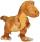More than 30 and less than 60 dinosaurs have met at the pond. A quarter of them bathed and 1/7 saws and the rest gripped. How many were at the pond? How many were there?
2. School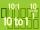Headteacher think whether the distribution of pupils in race in groups of 4,5,6,9 or 10. How many pupils must have at least school at possible options?
3. Apples 2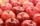How many minimum apples are in the cart, if possible is completely divided into packages of 6, 14 and 21 apples?
4. The smallest numberWhat is the smallest number that can be divided by both 5 and 7
5. Balls groups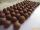Karel pulled the balls out of his pocket and divide it into the groups. He could divide them in four, six or seven, and no ball ever left. How little could be a ball?
6. LCMCommon multiple of three numbers is 3276. One number is in this number 63 times, second 7 times, third 9 times. What are the numbers?
7. Counting numberWhat is the smallest counting number divisible by 2,5,7,8 and 15?
8. Cents no more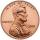Janko bought pencils for 35 cents each. Neither he nor the salesperson had small coins just a whole € 1 coin. At least how many pencils had to buy to pay for the whole euros?
9. ProductResult of the product of the numbers 1, 2, 3, 1, 2, 0 is:Added together and write as decimal number: LXVII + MLXIV
11. Lcm simpleFind least common multiple of this two numbers: 140 175.
12. The largest numberFind the largest integer such that: 1. No figures is not repeat, 2. multiplication of every two digits is odd, 3. addition all digits is odd.
13. Lcm of three numbersWhat is the Lcm of 120 15 and 5
14. Tram lines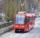Trams of five lines driven at intervals of 5,8,10,12 and 15 minutes. At 12 o'clock come out of the station at the same time. About how many hours again all meet? How many times have earch tram pass for this stop?
15. SickSick Marcel already taken six tablets, which was a quarter of the total number of pills in the pack. How many pills were in the pack?
16. Seeds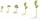Of the 72 wet seeds 7/8 germinated. How many seeds germinate?
17. MidpointsTriangle whose sides are midpoints of sides of triangle ABC has a perimeter 45. How long is perimeter of triangle ABC?